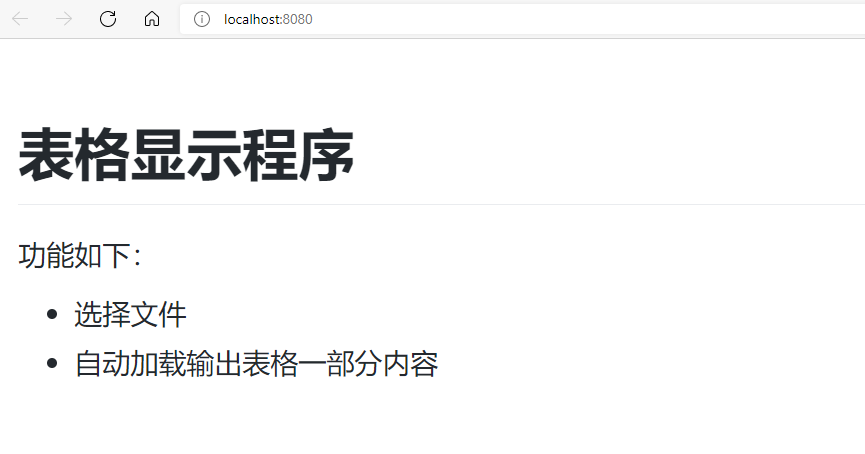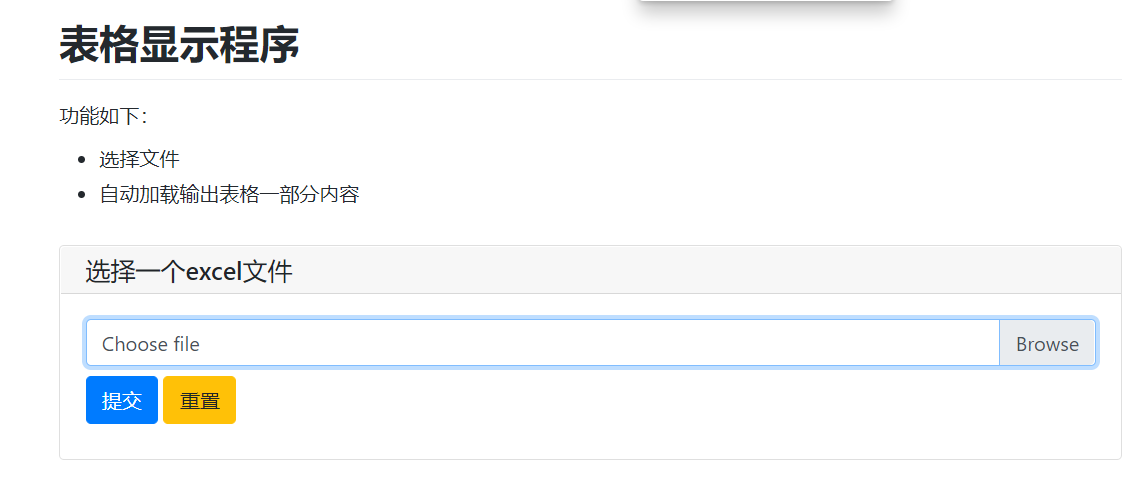﻿ Python PyWebIO实现网页版数据查询器_python_脚本之家
python# Python PyWebIO实现网页版数据查询器

PyWebIO提供了一系列命令式的交互函数来在浏览器上获取用户输入和进行输出，将浏览器变成了一个“富文本终端”，可以用于构建简单的Web应用或基于浏览器的GUI应用。本文将利用PyWebIO制作一个网页版的数据查询器，感兴趣的可以学习一下• 选择 excel 文件
• 输出文件第一个工作表的数据(DataFrame)

`pip install -U pywebio`

```import pywebio
import pywebio.output as output

def main():
output.put_html('表格显示程序')

if __name__=='__main__':
pywebio.start_server(main, port=8080, debug=True, cdn=False,auto_open_webbrowser=True)``````def main():
output.put_markdown('# 表格显示程序')
output.put_markdown('功能如下：')
output.put_markdown("""
- 选择文件
- 自动加载输出表格一部分内容
""")``````import pywebio
import pywebio.output as output
import pywebio.input as input

def main():
output.put_markdown('# 表格显示程序')
output.put_markdown('功能如下：')
output.put_markdown("""
- 选择文件
- 自动加载输出表格一部分内容
""")```import pywebio
import pywebio.output as output
import pywebio.input as input
import pandas as pd

def main():
output.put_markdown('# 表格显示程序')
output.put_markdown('功能如下：')
output.put_markdown("""
- 选择文件
- 自动加载输出表格一部分内容
""")```import pywebio
import pywebio.output as output
import pywebio.input as input
import pandas as pd

def main():
output.put_markdown('# 表格显示程序')
output.put_markdown('功能如下：')
output.put_markdown("""
- 选择文件
- 自动加载输出表格一部分内容
""")